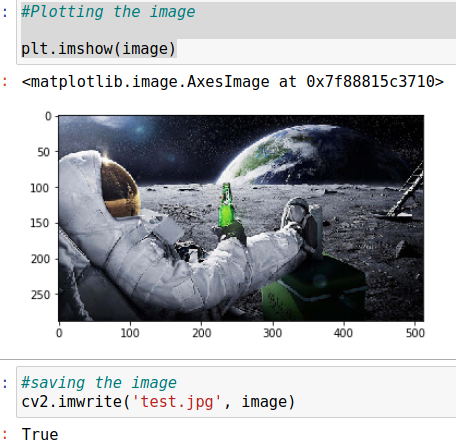# Image Processing in OpenCv - Part 1

03.09.20 01:00 PM

## Introduction Image Processing in OpenCv

In this article, you will learn Image Processing in OpenCv like how to read an image, how to display it and how to save it back

The below function is used for reading, show, and save images.

• cv2.imshow() :- show the images(display)
• cv2.imwrite() :- write the image

cv2.imread() to read an image.The image should be in the working directory or a full path of the image should be given.

Another argument is a flag which specifies the way image read should be,

cv2.IMREAD_COLOR:- (1) Loads a color image. Any transparency of the image will be neglected. It is the default flag.

NOTE: Instead of these three flags, you can simply pass integers 1, 0, or -1 respectively.

``````
Sample Code:
Import required Library

import numpy as np
import matplotlib.pyplot as plt
import cv2
%matplotlib inline

print(image)

Output:
array(,
,
,
...,
,
,
],

,
,
,
When read a image using OpenCV, and print it get a matrix in output. It means pixel of that image.

image = cv2.cvtColor(image, cv2.COLOR_BGR2RGB)
#Plotting the image
plt.imshow(image)

``````Now, the line of code is to show the original image using the cv2 function.

## Save an Image

With using of CV2 function, show the original image in python code. Rember that image is print out without CV function you will get pixel of that image in matrix form.

## Image Properties

``````print("Image Properties")
print("Number of Pixels:" + str(image.size))
print("Dimension of Image:" + str(image.shape))

Output:
Image Properties
Number of Pixels:442368
Dimension of Image:(288, 512, 3)
``````

Above function used to calculate the number of pixels has given image and dimension. We can see Number of pixels and Dimensions both are calculated is in str (string format).

## Conclusion

In this article, we discussed about Image Processing in OpenCv and understand the read and save it image using the CV2 library. Also, check the pixels and dimensions size.